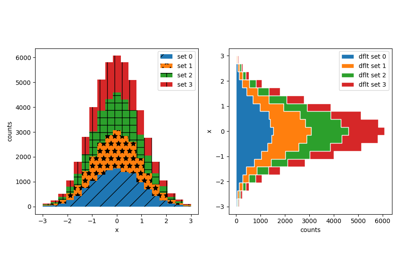# matplotlib.axes.Axes.fill_betweenx¶

Axes.fill_betweenx(y, x1, x2=0, where=None, step=None, interpolate=False, *, data=None, **kwargs)[source]

Fill the area between two vertical curves.

The curves are defined by the points (x1, y) and (x2, y). This creates one or multiple polygons describing the filled area.

You may exclude some vertical sections from filling using where.

By default, the edges connect the given points directly. Use step if the filling should be a step function, i.e. constant in between y.

Parameters:
y : array (length N)

The y coordinates of the nodes defining the curves.

x1 : array (length N) or scalar

The x coordinates of the nodes defining the first curve.

x2 : array (length N) or scalar, optional, default: 0

The x coordinates of the nodes defining the second curve.

where : array of bool (length N), optional, default: None

Define where to exclude some vertical regions from being filled. The filled regions are defined by the coordinates y[where]. More precisely, fill between y[i] and y[i+1] if where[i] and where[i+1]. Note that this definition implies that an isolated True value between two False values in where will not result in filling. Both sides of the True position remain unfilled due to the adjacent False values.

interpolate : bool, optional

This option is only relvant if where is used and the two curves are crossing each other.

Semantically, where is often used for x1 > x2 or similar. By default, the nodes of the polygon defining the filled region will only be placed at the positions in the y array. Such a polygon cannot describe the above semantics close to the intersection. The y-sections containing the intersecion are simply clipped.

Setting interpolate to True will calculate the actual interscection point and extend the filled region up to this point.

step : {'pre', 'post', 'mid'}, optional

Define step if the filling should be a step function, i.e. constant in between y. The value determines where the step will occur:

• 'pre': The y value is continued constantly to the left from every x position, i.e. the interval (x[i-1], x[i]] has the value y[i].
• 'post': The y value is continued constantly to the right from every x position, i.e. the interval [x[i], x[i+1]) has the value y[i].
• 'mid': Steps occur half-way between the x positions.
Returns:
.PolyCollection

A PolyCollection containing the plotted polygons.

Other Parameters:
**kwargs

All other keyword arguments are passed on to PolyCollection. They control the Polygon properties:

Property Description
agg_filter a filter function, which takes a (m, n, 3) float array and a dpi value, and returns a (m, n, 3) array
alpha float or None
animated bool
antialiased bool or sequence of bools
array ndarray
capstyle {'butt', 'round', 'projecting'}
clim a length 2 sequence of floats; may be overridden in methods that have vmin and vmax kwargs.
clip_box Bbox
clip_on bool
clip_path [(Path, Transform) | Patch | None]
cmap colormap or registered colormap name
color matplotlib color arg or sequence of rgba tuples
contains callable
edgecolor color or sequence of colors
facecolor color or sequence of colors
figure Figure
gid str
hatch {'/', '\', '|', '-', '+', 'x', 'o', 'O', '.', '*'}
in_layout bool
joinstyle {'miter', 'round', 'bevel'}
label object
linestyle {'-', '--', '-.', ':', '', (offset, on-off-seq), ...}
linewidth float or sequence of floats
norm Normalize
offset_position {'screen', 'data'}
offsets float or sequence of floats
path_effects AbstractPathEffect
picker None or bool or float or callable
pickradius unknown
rasterized bool or None
sketch_params (scale: float, length: float, randomness: float)
snap bool or None
transform Transform
url str
urls List[str] or None
visible bool
zorder float

fill_between
Fill between two sets of y-values.

Notes

Note

In addition to the above described arguments, this function can take a data keyword argument. If such a data argument is given, the following arguments are replaced by data[<arg>]:

• All arguments with the following names: 'where', 'x1', 'x2', 'y'.

Objects passed as data must support item access (data[<arg>]) and membership test (<arg> in data).

## Examples using matplotlib.axes.Axes.fill_betweenx¶Hatch-filled histograms